March 13, 2017

# Classification Algorithms

Algorithms that learn from labeled data how to classify new instances.

The following classification learning algorithm are divided into:

• Kernel Methods: methods operating in the Reproducing Kernel Hilbert Space
• Linear Methods: methods operating in the explicit primal space

# Kernel Methods

### Binary C SVM

Java classBinaryCSvmClassification

Source codeBinaryCSvmClassification.java

Maven Project: kelp-core

JSON type: binaryCSvmClassification

Description: It is the KeLP implementation of C-Support Vector Machine learning algorithm [Cortes and Vapnik(1995)]. It is a learning algorithm for binary classification and it relies on kernel functions. It is a Java porting of the library LIBSVM v3.17, written in C++ [Chang and Lin(2011)]

Parameters:

• kernel: The kernel function
• label: The label to be learned
• cp: The regularization parameter for positive examples
• cn: The regularization parameter for negative examples
• useFairness: A boolean parameter to force the fairness policy

### Binary ν-SVM

Java classBinaryNuSvmClassification

Source codeBinaryNuSvmClassification.java

Maven Project: kelp-core

JSON type: binaryNuSvmClassification

Description: It is the KeLP implementation of the ν-Support Vector Machine learning algorithm [Scholkopf et al.(2000)]. It is a learning algorithm for binary classification and it relies on kernel functions. It is a Java porting of the library LIBSVM v3.17, written in C++ [Chang and Lin(2011)].

Parameters:

• kernel: The kernel function
• label: The label to be learned
• nu: The ν parameter, i.e., the percentage of training example to be used as Support Vectors
• useFairness: A boolean parameter to force the fairness policy

### One-Class SVM

Java classOneClassSvmClassification

Source codeOneClassSvmClassification.java

Maven Project: kelp-core

JSON type: oneClassSvmClassification

Description: It is KeLP implementation of One-Class Support Vector Machine learning algorithm [Scholkopf et al.(2001)]. It is a learning algorithm for estimating the Support of a HighDimensional Distribution and it relies on kernel functions. The model is acquired only by considering positive examples. It is useful in anomaly detection (a.k.a. novelty detection). It is a Java porting of the library LIBSVM v3.17, written in C++ [Chang and Lin(2011)]

Parameters:

• kernel: The kernel function
• label: The label to be learned
• nu: The ν parameter, i.e., the percentage of training example to be used as Support Vectors

### Kernel-based Perceptron

Java classKernelizedPerceptron

Source codeKernelizedPerceptron.java

JSON type: kernelizedPerceptron

Description: The perceptron learning algorithm for classification tasks (Kernel machine version, presented in [Rosenblat(1958)]).

Parameters:

• kernel: The kernel function
• label: The label to be learned
• alpha: The learning rate, i.e., the weight associated to misclassified examples during the learning process
• margin: The minimum distance from the hyperplane that an example must have in order to be not considered misclassified
• unbiased: This boolean parameter determines the use of bias b in the classification function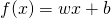. If usebias is set to true the bias is set to 0.

### Kernel-based Passive Aggressive (PA) Classification

Java classKernelizedPassiveAggressiveClassification

Source codeKernelizedPassiveAggressiveClassification.java

JSON type: kernelizedPA

Description: Online Passive-Aggressive Learning Algorithm for classification tasks with Kernels (presented in [Crammer et al.(2006)] and extended in [Filice et al.(2014)])). Every time an example is misclassified it is added as support vector, with the weight that solves the passive aggressive minimization problem.

Parameters:

• label: The label to be learned
• kernel: The kernel function
• loss: The loss function to weight each misclassification
• policy: The updating policy applied by the Passive Aggressive Algorithm when a miss-prediction occurs
• cp: The aggressiveness parameter for positive examples
• cn: The aggressiveness parameter for negative examples
• useFairness: A boolean parameter to force the fairness policy

### Budgeted Online Learning: Budgeted Passive Aggressive Classification

Java classBudgetedPassiveAggressiveClassification

Source codeBudgetedPassiveAggressiveClassification.java

JSON type: budgetedPA

Description: It is the implementation of the Budgeted Passive Aggressive Algorithm proposed in [Wang and Vucetic(2010)]. When the budget is full, the schema proposed in [Wang and Vucetic(2010)] to update examples (and weights) is adopted.

Parameters:

• label: The label to be learned
• label: The label to be learned
• kernel: The kernel function
• loss: The loss function to weight each misclassification
• policy: The updating policy applied by the Passive Aggressive Algorithm when a miss-prediction occurs
• deletingPolicy: The policy for the removal of examples from the budget before adding new examples. This can be
• BPA S: Budgeted Passive Aggressive Simple: when a new support vector must be added, one is removed and the weight of the other support vectors is kept unchanged
• BPA 1NN: Budgeted Passive Aggressive Nearest Neighbor: when a new support vector must be added, one is removed and the weight of its nearest neighbor is adjusted
• cp: The aggressiveness parameter for positive examples
• cn: The aggressiveness parameter for negative examples
• useFairness: A boolean parameter to force the fairness policy
• budget: The maximum number of support vectors allowed in the budget

# Linear Methods

### LibLinear SVM Classification

Java classLibLinearLearningAlgorithm

Source codeLibLinearLearningAlgorithm.java

JSON type: liblinear

Description: This class implements linear SVMs models trained using a coordinate descent algorithm [Fan et al.(2008)]. It operates in an explicit feature space (i.e., it does not rely on any kernel). This code has been adapted from the Java port of the original LIBLINEAR C++ sources.

Parameters:

• label: The label to be learned
• cp: The regularization parameter for positive examples
• cn: The regularization parameter for negative examples
• representation: The identifier of the representation to be considered for the training step

### Pegasos Classification

Java classPegasosLearningAlgorithm

Source codePegasosLearningAlgorithm.java

JSON type: pegasos

Description: It implements the Primal Estimated sub-GrAdient SOlver (PEGASOS) for SVM. It is a learning algorithm for binary linear classification Support Vector Machines. It operates in an explicit feature space (i.e., it does not rely on any kernel). Further details can be found in [Shalev-Shwartz et al.(2007)].

Parameters:

• label: The label to be learned
• lambda: The regularization coefficient
• iterations: The number of iterations required from the learning algorithm
• k: The number of examples k that PEGASOS exploits in its mini-batch learning approach
• representation: The identifier of the representation to be considered for the training step

### A Dual Coordinate Descent Learning Algorithm

Java classDCDLearningAlgorithm

Source codeDCDLearningAlgorithm.java

JSON type: dcd

Description: the KeLP implementation of Dual Coordinate Descent (DCD) training algorithm for a Linear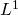or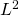Support Vector Machine for binary classification [Hsieh et al.(2008)].

Parameters:

• label: The label to be learned
• dcdLoss: The considered Loss function (or)
• useBias: This boolean parameter determines the use of bias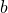in the classification function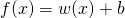. If usebias is set to false the bias is set to 0
• cp: The regularization parameter for positive examples
• cn: The regularization parameter for negative examples
• maxIterations: The number of iterations required from the learning algorithm
• representation: The identifier of the representation to be considered for the training step

### Linear Passive Aggressive (PA) Classification

Java classLinearPassiveAggressiveClassification

Source codeLinearPassiveAggressiveClassification.java

JSON type: linearPA

Description: Online Passive-Aggressive Learning Algorithm for classification tasks (linear version, presented in [Crammer et al.(2006)] and extended in [Filice et al.(2014)]). Every time an example is misclassified it is added the current hyperplane, with the weight that solves the passive aggressive minimization problem.

Parameters:

• label: The label to be learned
• loss: The loss function to weight each misclassification
• policy: The updating policy applied by the Passive Aggressive Algorithm when a miss-prediction occurs
• cp: The aggressiveness parameter for positive examples
• cn: The aggressiveness parameter for negative examples
• useFairness: A boolean parameter to force the fairness policy
• representation: The identifier of the representation to be considered for the training step

### Linear Perceptron

Java classLinearPerceptron

Source codeLinearPerceptron.java

JSON type:  linearPerceptron

Description: The perceptron learning algorithm for classification tasks (linear version, presented in [Rosenblat(1958)]).

Parameters:

• label: The label to be learned
• alpha: The learning rate, i.e., the weight associated to misclassified examples during the learning process
• margin: The minimum distance from the hyperplane that an example must have in order to be not considered misclassified
• unbias: This boolean parameter determines the use of bias b in the classification function. If usebias is set to true the bias is set to 0.
• representation: The identifier of the representation to be considered for the training step

### Soft Confidence Weighted Classification

Java classSoftConfidenceWeightedClassification

Source codeSoftConfidenceWeightedClassification.java

JSON type: scw

Description: Implements Exact Soft Confidence-Weighted (SCW) algorithms, an on-line learning algorithm for binary classification [Wang et al.(2012)]. This class implements both the SCW-I and SCW-II variants.

Parameters:

• label: The label to be learned
• scwType: The type of SCW learning algorithm (SCW-I or SCW-II)
• eta: The probability of correct classification required for the updated distribution on the current instance
• cp: The regularization parameter for positive examples
• cn: The regularization parameter for negative examples
• useFairness: A boolean parameter to force the fairness policy
• representation: The identifier of the representation to be considered for the training step

### References

Nicolo’ Cesa-Bianchi and Claudio Gentile. Tracking the best hyperplane with a simple budget perceptron. In Proc. Of The Nineteenth Annual Conference On Computational Learning Theory, pages 483–498. Springer-Verlag, 2006.

Koby Crammer, Ofer Dekel, Joseph Keshet, Shai Shalev-Shwartz, and Yoram Singer. On-line passive-aggressive algorithms. Journal of Machine Learning Research, 7:551–585, December 2006. ISSN 1532-4435.

R.-E. Fan, K.-W. Chang, C.-J. Hsieh, X.-R. Wang, and C.-J. Lin. LIBLINEAR: A library for large linear classification Journal of Machine Learning Research 9(2008), 1871-1874.

Hsieh, C.J., Chang, K.W., Lin, C.J., Keerthi, S. S. and Sundararajan, S. (2008). A Dual Coordinate Descent Method for Largescale Linear SVM. Proceedings of the 25th international conference on Machine learning ICML ’08 (pp. 408415). New York, New York, USA: ACM Press.

F. Rosenblatt. The Perceptron – a perceiving and recognizing automaton. Report 854601, Cornell Aeronautical Laboratory (1957)

S. Shalev-Shwartz, Y. Singer, and N. Srebro. Pegasos: Primal estimated sub–gradient solver for SVM. In Proceedings of the International Conference on Machine Learning, 2007.

Wang, J., Zhao, P. and Hoi, S.C.. Exact soft confidenceweighted learning. In Proceedings of the ICML 2012. ACM, New York, NY, USA (2012)

Zhuang Wang and Slobodan Vucetic. Online PassiveAggressive Algorithms on a Budget. JMLR W&CP 9:908-915, 2010